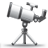## Are csc coefficients in the imx6 IPU v3 properly computed?

cancel
Showing results for
Did you mean:
SOLVED

## Are csc coefficients in the imx6 IPU v3 properly computed?

676 ViewsContributor V

This is Android code 13.4.1.04 for imx6 quad core chip. Funtion is _init_csc in kernel_imx/drivers/imx/ipu3. Matrix rgb2rgb_coeff has diagonal elements 0x80 and according to the code scale value is 2. Comparing with the chip spec chapter 37.4.5.6. IC Task Parameter Memory, scale value 2 means that 1/2 is applied to the coefficient. Therefore the final value is (0x80 * 1/2) / 256 = 0x40 / 256 = 0.25. I think that the intent was to apply 1 so the coefficient should be 0x80 but scaling should b be 0 which means that 2 is applied: (0x80 * 2) / 256 = 0x100 / 256 = 1.

Am I missing something?

Also, I'm not clear why are A1 and A2 in rgb2ycbcr_coeff coded as 0x0200 to get 128 as per the formula given in the code. Can you please explain me how we get 128 fro 0x200?

Thanks.

Labels (2)
• ### Android

Tags (4)
1 Solution
104 ViewsNXP Employee

Hi Dragan,

Question 1:

The scale for rgb2rgb is = 2, it means that the coeficient will be = 2 (as in manual formula: coeficient=2^(scale-1))

The final value will be: 0x80*2/256 = 1

Question 2:

The offset format is: sxxxxxxxxxx.xx -> where s = signal and x = binary number

on code, A = 0x0200 = 0000 0010 0000 0000

applying the format: 000s00 (10000000).00

The number between () will be 0x80 = 128d

Rgds

Rogerio

2 Replies
105 ViewsNXP Employee

Hi Dragan,

Question 1:

The scale for rgb2rgb is = 2, it means that the coeficient will be = 2 (as in manual formula: coeficient=2^(scale-1))

The final value will be: 0x80*2/256 = 1

Question 2:

The offset format is: sxxxxxxxxxx.xx -> where s = signal and x = binary number

on code, A = 0x0200 = 0000 0010 0000 0000

applying the format: 000s00 (10000000).00

The number between () will be 0x80 = 128d

Rgds

Rogerio

104 ViewsContributor V

Thanks Rogerio, now it's clear. Much appreciated for the answer.